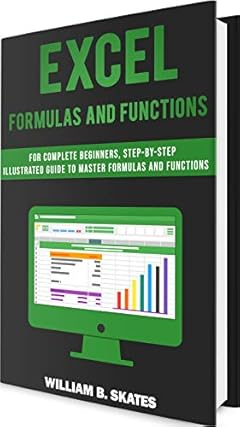# Excel Formulas and Functions: For Complete Beginners, Step-By-Step Illustrated Guide to Master Formulas and FunctionsView On Amazon
Excel Formulas and Functions: For Complete Beginners, Step-By-Step Illustrated Guide to Master Formulas and Functions
Author
: William B. Skates
Publisher
:
Pages
: 91
Category
: Non Fiction : General
View On Amazon Description

# Do you want to become an expert at Excel Formulas?

## You have come to the right place!

### In This Book You Will Learn:

• The fundamentals of Excel Formulas and Functions.
• The difference between Absolute and Relative Cell References
• What are comparison formulas
• What are Excel Operators
• How to use text formulas
• And more!

Do you struggle with Excel Formulas and Functions and want to learn more about how they work? If so, this is the book you've been looking for. Wether it be learning how to use VLOOKUP and HLOOKUP or simply comparing two columns with a formula, the goal of this book is provide you with an illustrated step-by-step guide that will help you learn more about Excel Formulas and Functions and the tech stuff that comes with it.Each of the chapters has examples to make the process of creating your own formulas as simple as possible. This book is not only to be used by beginners but also intermediates as a referencing tool when needed.

## Become an Expert in 20 Chapters

• Chapter 1: What are Formulas and Functions?

• Chapter 2: Text Formulas

• Chapter 3: Comparison Formulas

• Chapter 4: Operators

• Chapter 5: Absolute vs. Relative Cell References

• Chapter 6: SUM

• Chapter 7: IF

• Chapter 8: AND

• Chapter 9: LEN

• Chapter 10: OR

• Chapter 11: NOT

• Chapter 12: XOR

• Chapter 13: SUMIF and SUMIFS

• Chapter 14: COUNT and COUNTA

• Chapter 15: AVERAGEIF and AVERAGEIFS

• Chapter 16: LARGE and SMALL

• Chapter 17: COUNTIF and COUNTIFS

• Chapter 18: VLOOKUP

• Chapter 19: HLOOKUP

• Chapter 20: A few notes about pasting, ordering and filtering

View On Amazon
...